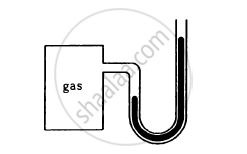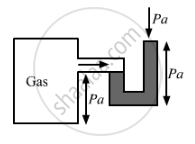Department of Pre-University Education, KarnatakaPUC Karnataka Science Class 11
Advertisement Remove all ads

# The Heights of Mercury Surfaces in the Two Arms of the Manometer Shown in Figure Are 2 Cm and 8 Cm - Physics

Answer in Brief

The heights of mercury surfaces in the two arms of the manometer shown in figure are 2 cm and 8 cm.
Atmospheric pressure = 1.01 × 105 N−2. Find (a) the pressure of the gas in the cylinder and (b) the pressure of mercury at the bottom of the U tube.Advertisement Remove all ads

#### Solution

(a) Given:
Height of the first arm, h1 = 8 cm
Height of the second arm, h2 = 2 cm
Density of mercury, ρHg = 13.6 gm/cc
Atmospheric pressure, pa = 1.01 × 105 N/m2 = 1.01 × 106 dyn/cm
Now,
Let pg be the pressure of the gas.
If we consider both limbs, then the pressure at the bottom of the tube will be the same.According to the figure, we have:
pg + ρHg × h2 × g = Pa + ρHg × hg

=> Pg =Pa +ρHg × g(h1-h2)

=1.01 ×106+13.6 ×980 ×(8-2) dyn/cm2

=(1.01 ×106+13.6 ×980 ×6)dyn/cm2

= 1.09 × 105N/m2

(b) Pressure of the mercury at the bottom of the U-tube:

PHg =Pa + ρHg×h1×g

=1.01×106+13.6×8×980

=1.12×105N/m2

Is there an error in this question or solution?
Advertisement Remove all ads

#### APPEARS IN

HC Verma Class 11, 12 Concepts of Physics 1
Chapter 13 Fluid Mechanics
Q 2 | Page 273
Advertisement Remove all ads
Advertisement Remove all ads
Share
Notifications

View all notifications

Forgot password?